MORE SITES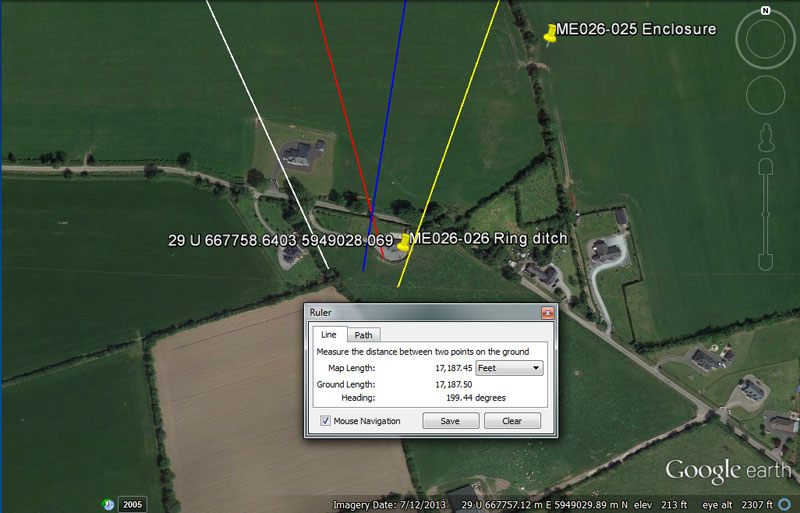The archaeological register places a ring ditch at the coordinates shown within Gillinstown.

From Knowth = 15360’ @ 155.52-degrees.

This distance is navigational coding and 153.6-miles would be 1/162nd of the 24883.2-mile equatorial circumference, whereas 1.536-miles would be 1/45th of 1-degree of arc.

The degree angle is dynamic navigational coding and 1555.2-miles would be 1/16th of the 24883.2-mile equatorial circumference.

From Newgrange = 12000’ @ 165-degrees.

This distance is self-explanatory.

The degree angle is in homage to the rod or perch increment @ 16.5' (3 ancient fathoms) or the league @ 16500'.

From Dowth Mound = 15120’ @ 189-degrees.

Again a repeat of the popular 15120' increment or 2.5-minutes of arc.

Again a repeat of the 189 value, used in navigational or lunar calculations.

From Dowth Henge = 17187.5’ @ 19.44-degrees.

Again a repeat of the 17187.5' length. The distance is navigational coding and 171.875-miles (171 & 7/8ths) would be 1/144th of the 24750-mile circumference or 2.5-degrees of arc. The distance of 17187.5' would be 10,000 Egyptian Royal cubits of 20.625" each.

The degree angle is navigational coding also and 194.4-miles would be 1/128th of the 24883.2-mile circumference.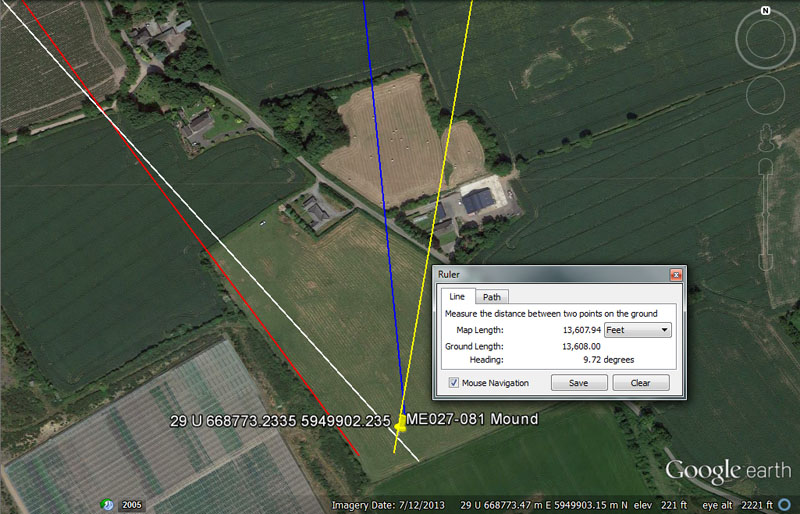The archaeological register places a ploughed over mound at these coordinates at Corballis (Duleek Lower By.)

From Knowth = 15120’ @ 138.24-degrees.

Again, the distance is 2.5-minutes of arc.

The degree angle is navigational in a dynamic way and 1382.4-miles is 1/18th of the 24883.2-mile equatorial circumference.

From Newgrange = 11000’ @ 324-degrees.

This distance is 2/3rds of a league of 16500'.

The degree angle code is multi-functional and can be applied to many kinds of calculations. In the 25920-year duration of the Precession of the Equinoxes, the sum of 3240-years would be 1/8th part. There would be 21 intervals of 324-days in the 6804-day lunar nutation cycle. The distance of 32.4-miles would be 1/768th of the 24883.2-mile equatorial circumference and 324' would be 1/15th of the 4860' mile, etc.

From Dowth Mound =  12150’ @ 354.375-degrees.

The length of 12150' is both lunar and navigational coding and there would be 56 intervals of 121.5-days in the 6804-day lunar nutation cycle (which might explain the 56-posts in the Aubrey Circle at Stonehenge). Likewise, there are 21 intervals of 121.5-days in the 2551.5-days (7.2 lunar years) monitored alongside 7-solar years in the lunisolar Sabbatical Calendar count. A length of 1215' is 1/4th of the 4860' mile and 12150' is 2.5 miles of 4860'.

The degree angle is in homage to the 354.375-day lunar year.

From Dowth Henge = 13608’ @ 97.2-degrees.

The 13608' (6804 X 2) distance is in homage to the 6804-day lunar nutation cycle.

The degree angle is navigational, as well as lunar coding and 97.2-miles would be 1/256th of the 24883.2-mile circumference, whereas 972' would be 1/5th of the 4860' mile. Alternatively, there would be 7 intervals of 972-days in the 6804-day lunar nutation cycle.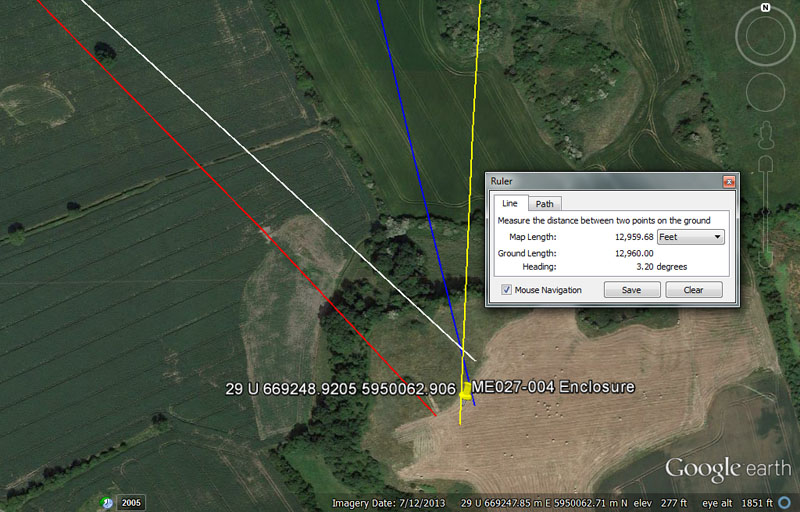An enclosure is registered to have been built atop this elevated Newtown bluff and students from the four centres could have found the structure by navigating according to the following distances and angles.

From Knowth = 15750 @ 312.5-degrees return.

This distance is, of course, 3 miles of 5250'.

The angle code relates to a version of the PI ratio rendered as 3.125 to 1 in several ancient calculations. For example, 7920-miles (the diameter of the Earth) X 3.125 = 24750-miles. Likewise, the mile of 5280' X 3.125 = 16500' (the English league). There were, as well, more accurate versions of PI used in navigation at sea, like 22/7ths or 1728/550ths. The sum of 3.125-miles is 1/22nd of 1-degree of arc (68.75-miles) under the 24750-mile equatorial circumference.

From Newgrange = 11664’ @ 136.08-degrees.

This distance is 2.4 so-called Roman miles. The Roman foot was 11.664". The Egyptian Theban volume was 11664 cubic inches, whereas their Besha was 1166.4 cu" and Honnu 116.64 cu".

The degree angle is in homage to the 6804-day lunar nutation cycle (136.08 ÷ 2 = 68.04).

From Dowth Mound = 12000’ @ 166.6666-degrees.

The distance code is self-explanatory.

The degree angle code is simply 166 & 2/3rds or 1/3rd of 500. In any 3,4,5 triangle one side will be 1.66666 less in length than the hypotenuse.

From Dowth Henge = 12960’ @ 3.2-degrees.

The distance codes the half value of the Precession of the Equinoxes duration in years. Thus 25920 ÷ 2 = 12960. likewise, there would be 1296000 seconds of arc (100.8') in the 24883.2-mile (of 5250') equatorial circumference.

The degree angle code is reminiscent of dividing the 360-degree compass into 32 or 320 parts, which has been standard practice since time immemorial.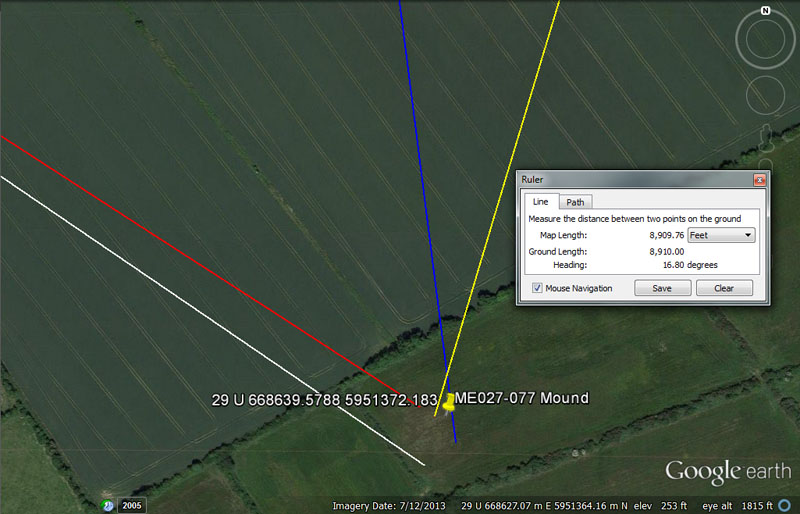This mound is still visible at Roughgrange.

From Knowth = 11664’ @ 303.75-degrees.

Again, 2.4 so-called Roman miles.

The degree angle also has a coded relationship to the 4860'-mile and 303.75' is 1/16th of that length.

From Newgrange = 7425’ @ 302.5-degrees.

The distance of 7425-miles would be .3 of the 24750-mile equatorial circumference. A length of 74250' would be 1/1760th of the 24750-mile equatorial circumference. A distance of 742.5' would equate to 1/8th of the ancient Scottish mile @ 5940', whereas 7425' would be 1.25 Scottish miles.

The degree angle is in homage to 3025' or 3024', both of which represent half a minute of equatorial arc under the two main navigational methods used by the great civilisations of antiquity.

From Dowth Mound = 7425’ @ 172.8-degrees.

Both Dowth and Newgrange chambered mounds lie equidistant from this field mound at Roughgrange and share the same distance coding.

The value 1728 (12 X 12 X 12) was very important to navigational and Precession of the Equinoxes calculations. In the 24883.2-mile circumference reading the sum of 172.8-miles would be 1/144th part. In the 25920-year duration of the Precession of the Equinoxes, 1728-years would be 1/15th part.

From Dowth Henge = 8910’ @ 16.6666 - 16.8-degrees (could also be comfortably read as 196.875-degrees).

This distance is, of course, 1.5 miles of 5940'.

The return degree angles could be read as 16 & 2/3rds or 16.8-degrees and both would have been sufficient for student navigators to locate the Roughgrange mound. Alternatively, an outrunning angle of 196.875-degrees (coding the Beqa grain weight for gold assaying) would have put students on a direct line to the mound.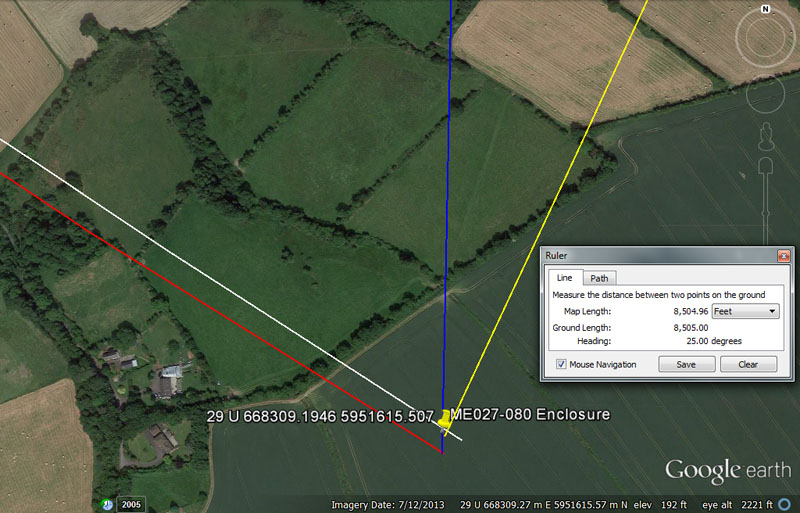Again at Roughgrange is this former enclosure, now reduced to a crop mark

From Knowth = 10368’ @ 302.4 or 302.5-degrees return.

This distance encodes the rate at which the Earth spins, thus 24883.2-miles ÷ 24-hours = 1036.8 MPH. Also, the distance of 10368' equates to 1/12600th of the 24883.2-mile circumference.

Again the angle code is navigational and both 3024' or 3025' represent half a minute of equatorial arc under the two main navigational methods used by the great civilisations of antiquity. The Great Pyramid @ 756' per side is 3024' for 1 circumnavigation. If the length was considered to be 756.25', then a circumnavigation would be 3025' and the second navigational method would come into play.

From Newgrange = 6180.34’ – 6187.5’ @ 121.5-degrees.

The distance is for mnemonic recall of the PHI reciprocal (.618034). Ancient assayers used the formula: 10" ÷ 1.6180339 = 6.18034". Increases of this length value were then used for setting out the diameters of the round bases for dry-goods tubs, fabricated for exact for measures of grain, etc., to be dispensed in the marketplace. By using this increment in diameters, one could square the circle such that within the walled and finished tub, code-bearing, perfect cubic inch capacities were achievable.

By the use of this formula for the fabrication of marketplace tubs, capacities were precise and no-one was being sold short measures. Inspectors or judges at the marketplaces, traditionally, have been very vigilant in assuring no-cheating or defrauding of the public, and in severe cases (in Scandinavia), repeat offenders could face the death penalty.
Other short-measure merchants could spend a few days locked in the marketplace stocks, with passers-by pelting them with rotten vegetables. Such penalties paved the way for the Baker's Dozen (13 instead of 12), where an extra bun was included free of charge or cubic capacity quantities were topped up to exceed the standard measure.
Few things in society caused more discontent and disruption to the public peace than being constantly short-changed at the marketplaces by thieving merchants. If people bought a bushel of grain, they wanted nothing less than a bushel of grain.

Again, 1215' would equate to 1/4th of a so-called Roman mile of 4860' and the 1215-value was highly important to several types of calculations.

From Dowth Mound = 6534’ @ 1.25 – 1.26-degrees (also 181.44-degrees).

The distance is navigational and 6534' X 2 = 13068'. In the 24750-mile (of 5280') equatorial circumference there are 130680000', so this distance (6534') equates to 1/20000th of the equatorial circumference under that navigational assignment.

The return angle codes @ 1.25 or 1.26-degrees are fairly self explanatory. What became the Greek foot was 12.6" long and the Hebrew reed was 126". A coded, outrunning angle of 181.44-degrees would also have placed Dowth Henge students onto the assigned objective. Twice 181440' would be 362880' or the number of feet in 1-degree of arc for a world of 24883.2-miles (of 5250' each).

From Dowth Henge = 8505’ @ 25-degrees return.

This is, of course, lunar coding and 850.5-days would be 1/8th of the 6804-day lunar nutation cycle.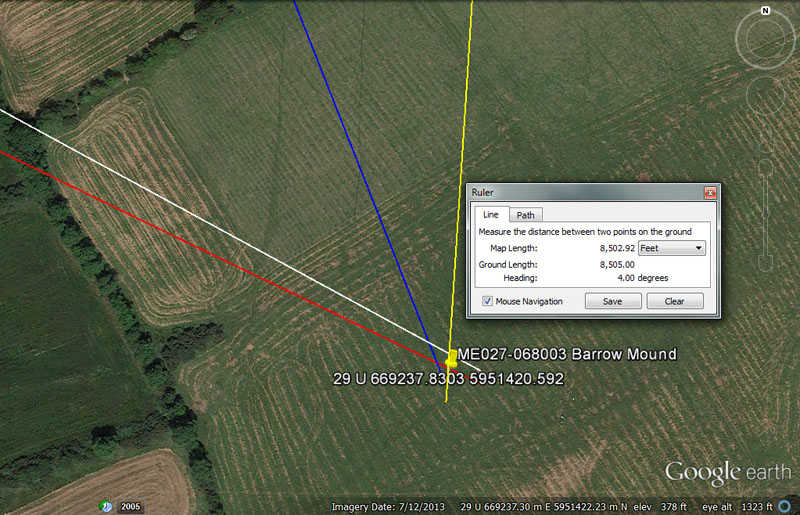On Stalleen Hill the ancient architect-astronomer-navigators set up 3 close-proximity coded positions that would have been visible for miles and certainly from some or all of the 4 main centres on the other side of the Boyne River.

From Knowth = 13333.3333 @ 118.125-degrees.

This distance is 1/3rd of 40000'.

The degree angle value, rendered in days, would equate to 4 lunar months.

From Newgrange = 9216’ @ 295.3125-degrees.

The distance coding is navigational and 921.6-miles would be 1/27th of the 24883.2-mile circumference.

The degree angle codes the lunar month @ 29.53125-days (29 & 17/32nds). The ancient method for finding this refined number was 1890 ÷ 64.

From Dowth Mound = 7776’ @ 158.4-degrees.

The distance coding is navigational and 777.6-miles would be 1/32nd of the 24883.2-mile circumference. Under the ancient Sumerian-Babylonian weights system (light standard) 7776-grains was 1 Mina. Alternatively, under their double or heavy standard their Mina was 15552-grains or 7776 X 2.

The angle code is navigational also and 15840' would be 3-miles of 5280'. Alternatively, 1584-miles would be 1/5th of the 7920-mile diameter of the Earth.

From Dowth Henge = 8505’ @ 4-degrees return.

Again, coding for the lunar nutation cycle in this length.

The degree angle is self-explanatory.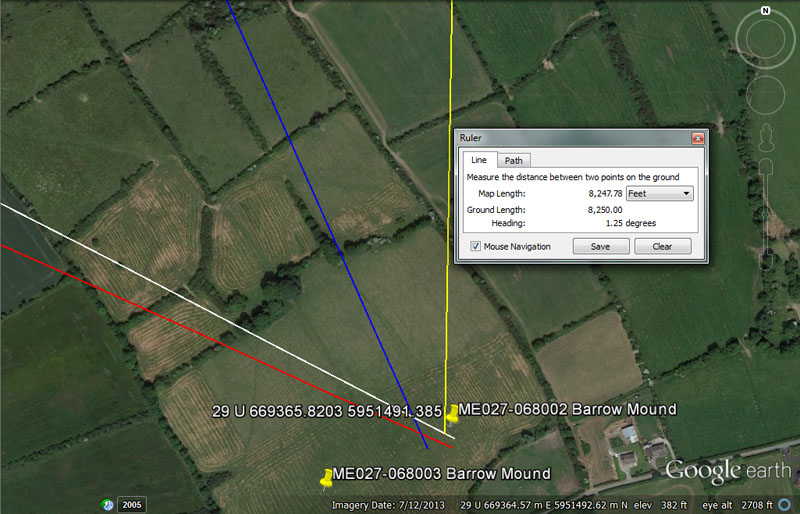Nearby on Stalleen Hill is this second barrow mound site.

From Knowth = 13608’ @ 116.64-degrees.

Again, coding related to the 6804-day lunar nutation cycle (13068 ÷ 2).

The angle is coding the so-called Roman foot @ 11.664" or the 11664' in 2.4 so-called Roman miles.

From Newgrange = 9504’ @ 113.4-degrees.

The sum of 95040' (18-miles of 5280' each) would be 1/ 1375th part of the 24750-mile circumference.

Again, the angle is lunar and encodes the lunar number 1134 and there would be 6 intervals of 1134-days in the lunar nutation cycle of 6804-days, etc, etc. This was one of the most important calculation values of antiquity.

From Dowth Mound = 7776’ @ 155.52-degrees.

Again, 777.6-miles is 1/32nd of the 24883.2-mile circumference.

Again 1555.2 is twice 777.6.

From Dowth Henge = 8250’ - 8294.4 @ 181.44-degrees. Also, 8294.4’.

Again, half a league in the 8250' distance. If read as 8294.4', then it would be coding for the equatorial size of the 24883.2-mile Earth, of which 8294.4-miles would be 1/3rd or 120-degrees of arc.

Again, the outrunning angle relates to the 362880' in 1-degree of arc, of which 181440' would be the half value.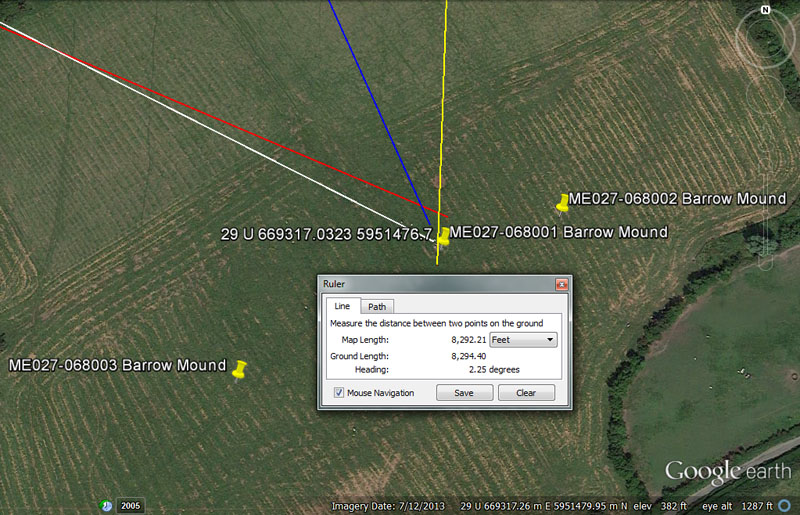And here's the third site on Stalleen Hill.

From Knowth = 13440’ @ 297-degrees.

This distance is 2 ancient Irish miles.

The degree angle mnemonically recalls the ancient Scottish mile of 5940' (2970' X 2).

From Newgrange = 93312’ @ 113.4-degrees.

This distance encodes a much used value of antiquity that found its way into the weights and measures standards of several civilisations. For example, the Sumerian / Babylonian heavy Talent was 933120-grains. The sum of 933120' would be 1/140th of the 24883.2-mile (130636800') equatorial circumference of the Earth.

Again, the very popular 1134 code in this degree angle.

From Dowth Mound = 7680’ @ 336-degrees.

The sum of 7680' would be 1/17010st of the 130636800' equatorial circumference. Of course, 1701-days would be 1/4th of the lunar nutation cycle. In the 69.12-mile reading for 1-degree of equatorial arc. the sum of 7.68-miles would be 1/9th part.

The degree angle relates to the ancient Irish mile (6720'), of which 3360' would be the half value.

From Dowth Henge = 8294.4’ @ 2.25-degrees.

Again, 8294.4-miles would equate to 1/3rd of the 24883.2-mile equatorial circumference.

The degree angle is self-explanatory as 160th of 360-degrees.

Continue